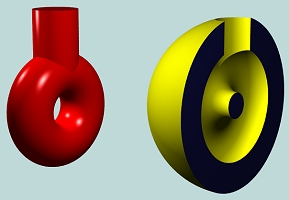# The Euler-Poincaré FormulaThe Euler-Poincaré formula describes the relationship of the number of vertices, the number of edges and the number of faces of a manifold. It has been generalized to include potholes and holes that penetrate the solid. To state the Euler-Poincaré formula, we need the following definitions:

• V: the number of vertices
• E: the number of edges
• F: the number of faces
• G: the number of holes that penetrate the solid, usually referred to as genus in topology
• S: the number of shells. A shell is an internal void of a solid. A shell is bounded by a 2-manifold surface, which can have its own genus value. Note that the solid itself is counted as a shell. Therefore, the value for S is at least 1.
• L: the number of loops, all outer and inner loops of faces are counted.
Then, the Euler-Poincaré formula is the following:

V - E + F - (L - F) - 2(S - G) = 0### Examples

• A cube has eight vertices (V = 8), 12 edges (E = 12) and six faces (F = 6), no holes and one shell (S=1); but L = F since each face has only one outer loop. Therefore, we have

V-E+F-(L-F)-2(S-G) = 8-12+6-(6-6)-2(1-0) = 0

• The following solid has 16 vertices, 24 edges, 11 faces, no holes, 1 shell and 12 loops (11 faces + one inner loop on the top face). Therefore,

V-E+F-(L-F)-2(S-G) = 16-24+11-(12-11)-2(1-0)=0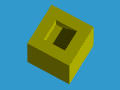• The following solid has 16 vertices, 24 edges, 10 faces, 1 hole (i.e., genus is 1), 1 shell and 12 loops (10 faces + 2 inner loops on top and bottom faces). Therefore,

V-E+F-(L-F)-2(S-G) = 16-24+10-(12-10)-2(1-1)=0• The following solid has a penetrating hole and an internal cubic chamber as shown by the right cut-away figure. It has 24 vertices, 12*3 (cubes) = 36 edges, 6*3 (cubes) - 2 (top and bottom openings) = 16 faces, 1 hole (i.e., genus is 1), 2 shells and 18 loops (16 faces + 2 inner loops on top and bottom faces). Therefore,

V-E+F-(L-F)-2(S-G) = 24-36+16-(18-16)-2(2-1)=0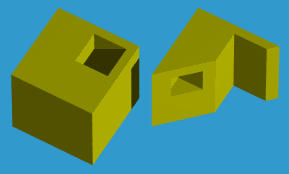• The following solid has two penetrating holes and no internal chamber as shown by the right cut-away figure. It has 24 vertices, 36 edges, 14 faces, 2 hole (i.e., genus is 2), 1 shells and 18 loops (14 faces + 4). Therefore,

V-E+F-(L-F)-2(S-G) = 24-36+14-(18-14)-2(1-2)=0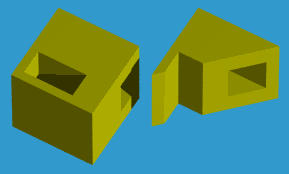Part of the information recorded in a B-rep is topological (i.e., adjacency relations). Invalid solids may be generated if the representation is not carefully constructed. One way of checking this topological invalidity is to use the Euler-Poincaré formula. If its value is not zero, we are sure something must be wrong in the representation. However, this is only a one-side test. More precisely, a zero value of the Euler-Poincaré formula does not mean the solid is valid.The figure above has a box and an additional sheet which is simply a rectangle. This object has 10 vertices, 15 edges, 7 faces, 1 shell and no hole. Its loop number is equal to the number of faces. The value of the Euler-Poincaré formula is zero as shown below,

V-E+F-(L-F)-2(S-G) = 10-15+7-(7-7)-2(1-0)=0

but this is not a valid solid! Therefore, if the value of Euler-Poincaré formula is non-zero, the representation is definitely not a valid solid. However, the value of the Euler-Poincaré formula being zero does not guarantee the representation would yield a valid solid.

### How to count genus correctly?

It is not always easy to count genus G correctly. For example, suppose we have a sphere punched by three tunnels as shown below. The left object shows the outside look. The right one shows the inside by cutting half of the sphere off. What is the genus value G? We know that the genus value counts the number of penetrating holes. But, in this example, it is somewhat ambiguous. In fact, by combining any two center-going tunnels we will have three penetrating holes. However, this is incorrect!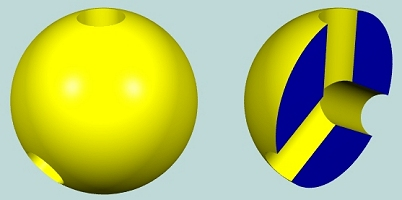The Euler-Poincaré Formula describes the topological property amount vertices, edges, faces, loops, shells and genus. Any topological transformation applied to the model will not alter this relationship. Intuitively, applying topological transformations means we can twist, stretch and squash the model but we cannot cut some parts off nor glue some parts together. Let us apply some intuitive topological transformations to this model for computing the genus. We can push the "walls" surrounding the three tunnels so that the interior of the model becomes a thin shell. This is shown in the image below.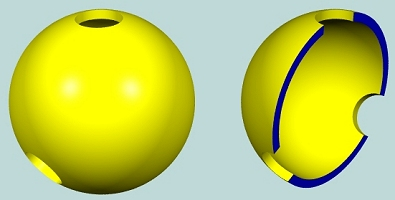Stretch the top hole so that it is large enough (below left). Then, collapse the top portion to flatten the model. This is shown in the right model below. How many penetrating holes are there? Two! Therefore, G is 2.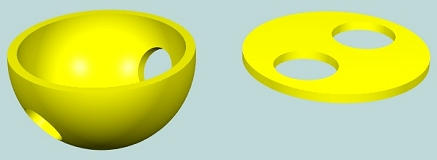Sometimes, penetrating holes may appear in an unlikely situation. Consider the following model which is obtained by taking out a torus and tube from the interior of a sphere. What is the genus of this model? It does not look like there is a penetrating hole. So, is genus equal to 0? In fact, G = 1! Figure it out yourself. Do some twisting, stretching and squashing.